# Horizontal and vertical analysis: step-by-step instructions on how to do it and why it is used

Define ―both horizontal and vertical analysis: step-by-step instructions on how to do it and why it is used. Please give two numerical examples for each State if it has improved, deteriorated, or unchanged. Critique your class member’s calculations, interpretations, and conclusions.

This post was part of TyroCity discussion forum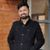Angel Paudel

Horizontal analysis can be performed by comparing a recent year against the base year while identifying the growth trends between the time periods. The analysis can be performed in any four types of financial statement i.e. income statement, balance sheet, statement of cash flow, and statement of changes in equity. However, income statement and balance sheet are mostly used financial statement to do horizontal analysis (Lubatkin, 1983). And, that is what we’re going to base the post on.

To do horizontal analysis, these are the steps that one needs to follow through:

• Calculate for the comparing year index. This is done by doing comparing year/base year.
• Once the index is calculated for the comparing year, multiply the result by 100 and then subtract it by the base year index.
• Once the result is out showing either the increase of decrease, find out why the result is so. This need to be done even when the result is positive.

Horizontal analysis is used by companies to see what has been the factors to drive the company’s financial performance over a number of years (Aizenman & Marion, 2004). With the use of this analysis, companies can also identify growth pattern and spot trends like during hurricane in the United States, the demand of water bottles is higher so Walmart stocks more of it as per the prediction that hurricane is going to occur. (Miller & Goidel, 2009) Like in Nepal as well, the demand/sell of clothes and other appliances is higher during special festivals or occasions compared to other normal days. It allows the company to have a detailed look at each of the line item. They can even have a complete picture of an operational result by analyzing financial statement, balance sheet, and cash flow statement at the same time. With it, the company can assess its profitability and operational efficiency while also looking at what has been driving the company’s performance.

Let’s look at an example of horizontal analysis using balance sheet first. It includes three main components i.e. assets, liabilities and equity. An example of a company’s asset can be a building; liability can be a loan on the building, and equity can be the total investment in the company. Let’s look at the assets of a sample company to look at the horizontal analysis of the same: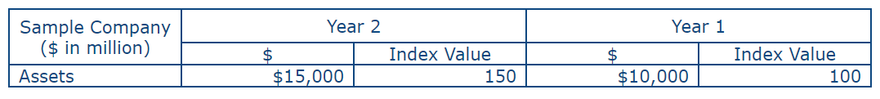As in the table, year 1 is considered to be base and its index value is thus taken to be 100. For Year 2 to calculate the index value we divide the dollar value of it with the dollar value in year 1 and multiply it by 100, which is as below:

(15000/10000) * 100 = 1.5 * 100 = 150

Now, to compare the index value between two years, it’s 150 - 100 = 50. Through this, we can say that the assets increased from year 1 to year 2 by 50%. The company should look into why it rose by 50% for this discussion with the accounting department can be scheduled to know what assets were purchased in year 2 to give it a significant rise.

Taking another example using the income statement of whose sales revenue is taken into account. This helps in calculating the profit/loss from the overall operation. The calculation is as follows: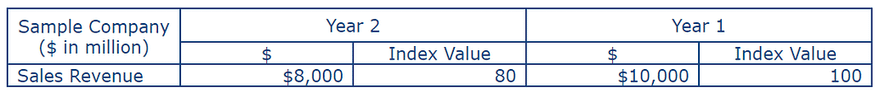Year 1 sales revenue is considered to be base and thus has its index value as 100. For year 2, to calculate the index value, we follow the same procedure as done above:

(8000/10000) * 100 = 0.8 * 100 = 80

Now, to compare the index value of year 2 and 1, we get 80 - 100 = -20. We can see that sales revenue actually took a dive in year 2. We need to determine why for this as well and thus look into what made it happen. Like did the competitors had extensive marketing campaigns? Was the advertisement sent out by the company not effective/enough? And other questions to get to the root of it.

Vertical analysis is when different aspects of the financial statement are compared in terms of percentage of the total amount (Amihud & Lev, 1981). An example of this can be when you bought a car for say \$50,000 and started comparing how much you paid for different parts of the car. You figured that the engine cost \$5,000, you can say that it cost you 10% of the total amount. This type of analysis is called as vertical analysis. Like horizontal analysis, it is also compared usually on the income statement and balance sheet. We’re often looking at the percentage for this analysis. With this analysis, we can see where the money is going and if it’s time to make an investment on a new technology, find an alternative supplier, reallocate cash or make the adjustment to inventory.

To do vertical analysis, these are the steps that one needs to follow through:

• For the balance sheet, the items of the sheet are divided by total assets. After which they’re multiplied by 100 to get a percentage value.

• For the income statement, the items of the statement are divided by revenue. After which they’re multiplied by 100 to get a percentage value.

• Perform analysis on the result regardless of it showing a positive or negative outcome on why that happened.

With vertical analysis, one can see the relative proportions of account balance. This simplifies the process of comparing the financial statement of the company against another or to even do it across the industry. This analysis also gives a better picture of the performance metrics of the company and if it’s improving or on a decline. That is done by looking at the annual or quarterly figures of the company and comparing it over a number of years.

An example of vertical analysis considering the balance sheet (simplified version for demonstration) is as below: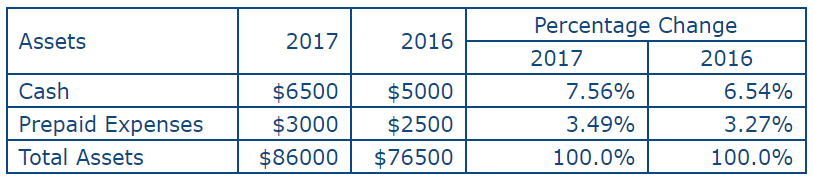In the above table, following calculations are made and populated:

Percentage change for Cash for 2017 = (6500/86000)*100 = 7.56%

Percentage change for Cash for 2016 = (5000/76500)*100 = 6.54%

Percentage change for Prepaid Expenses for 2017 = (3000/86000)*100 = 3.49%

Percentage change for Prepaid Expenses for 2016 = (2500/76500)*100 = 3.27%

From the analysis, we can make out that both cash and prepaid expenses increased in 2017 compared to 2016.

Similarly, looking at another example with select parameters taken into account from the financial statement is as below: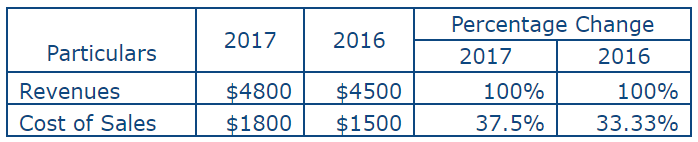The value in the above table is populated after the following calculation where the percentage change is computed by dividing the item (cost of sales) by revenue as this:

Percentage of Cost of Sales for 2017 = (1800/4800)*100 = 37.5%

Percentage of Cost of Sales for 2016 = (1500/4500)*100 = 33.33%

The calculated percentage value is used in the table above. The following analysis shows that the portion of the cost of sales has increased by over 4% comparing the records of 2017 and 2016.

References

Aizenman, J., & Marion, N. (2004). The merits of horizontal versus vertical FDI in the presence of uncertainty. Journal Of International Economics , 62 (1), 125-148.

Amihud, Y., & Lev, B. (1981). Risk Reduction as a Managerial Motive for Conglomerate Mergers. The Bell Journal Of Economics , 12 (2), 605.

Lubatkin, M. (1983). Mergers and the Performance of the Acquiring Firm. Academy Of Management Review , 8 (2), 218-225.

Miller, A., & Goidel, R. (2009). News Organizations and Information Gathering During a Natural Disaster: Lessons from Hurricane Katrina. Journal Of Contingencies And Crisis Management , 17 (4), 266-273.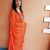Horizontal Analysis

Horizontal analysis takes into consideration the financial statements of different years and analyzes how individual items in the financial statements has changed over time. It is also known as trend analysis or time series analysis as it focuses on changes of variables in different accounting years. It helps us to detect trends, patterns, cyclicality and compare those factors with the organizations of similar nature to understand the current position of the firm (John, 2017). The advantage of horizontal analysis is it expresses change in both amounts and percentage and facilitates the intra-company comparison. It helps to see how each item has changed with respect to change in other items. The steps in performing horizontal analysis are:

Calculate the absolute change by deducting amount of base (previous) year from the amount of comparing year.

Calculate the percentage change by dividing the absolute change by amount of base year and multiplying the result by 100.

Interpret the result.

Vertical Analysis

In vertical analysis, the relationship between different variables during an accounting period is measured. In this method, each item is expressed as a percentage of total (sales in case of income statement and total assets in case of balance sheet). It is used to determine the relationship between different variables of financial statement (Singh, 2016). The steps in vertical analysis are:

Assume sales or total assets as 100%.

Calculate the percentage of each item as a percentage of sales or total assets but dividing the amount of the selected item with sales/total assets and multiplying it by 100.

Interpret the result.

Example

Let us consider the following balance sheet for horizontal and vertical analysis:

Balance Sheet of XYZ Company for 2016 and 2017

2017 2016
Liabilities and Equity
Accounts Payable 307 303
Notes Payable 26 119
Other Current Liabilities 1,662 1,353
Total Current Liabilities 1,995 1,775
Long-term Debt 843 1,091
Common Stock 2,556 2,167
Total Liabilities and Equity 5,394 5,033
Assets
Cash 696 58
Account Receivable 956 992
Inventory 301 361
Other Current Assets 303 264
Total Current Assets 2,256 1,675
Net Fixed Assets 3,138 3,358
Total Assets 5,394 5,033

Horizontal Analysis

2017 (A) 2016 (B) Absolute Change Percentage Change
Liabilities and Equity C=A-B C/B*100
Accounts Payable 307 303 4 1.32
Notes Payable 26 119 -93 -78.15
Other Current Liabilities 1,662 1,353 309 22.84
Total Current Liabilities 1,995 1,775 220 12.39
Long-term Debt 843 1,091 -248 -22.73
Common Stock 2,556 2,167 389 17.95
Total Liabilities and Equity 5,394 5,033 361 7.17
Assets
Cash 696 58 638 1100.00
Account Receivable 956 992 -36 -3.63
Inventory 301 361 -60 -16.62
Other Current Assets 303 264 39 14.77
Total Current Assets 2,256 1,675 581 34.69
Net Fixed Assets 3,138 3,358 -220 -6.55
Total Assets 5,394 5,033 361 7.17

Vertical Analysis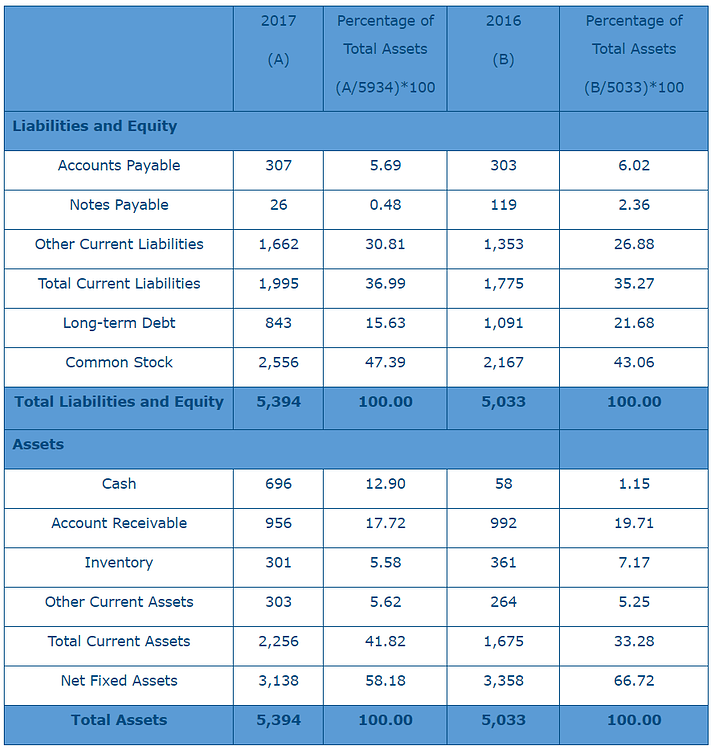From the above calculation, we can see that the account payables, total current liabilities, common stock, total current assets, cash has increased in the year 2017 while long-term debt and net fixed assets has decreased. The significant increase in cash is due to the collection of account receivable, issue of common stock, sale of goods and fixed assets. However the company is not utilizing the cash to meet the current liabilities which is not good for the business.

Similarly, the result of vertical analysis shows that the accounts payable, notes payable, account receivable, long-term debt, inventory and net fixed assets expressed in terms as percentage of total assets has lower value in 2017 than in 2016. On the other hand, total current liabilities, common stock, total current assets and cash has increased value. This indicates the company is performing well but it should use the cash in settling the current liabilities or invest it to maximize the return.

The analysis of the different items in income statement is also done following the similar procedure.

References

John. (2017, March 15). How to Perform Horizontal and Vertical Analysis of Income Statements. Retrieved from Vintage Value Investing: vintagevalueinvesting.com/perform-... 4

Singh, U. (2016, April). Analysis of Financial Statement. International Journal of Recent Research in Commerce Economics and Management (IJRRCEM), 3 (2), 1-10.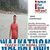Financial statement (Balance Sheet and Profit and Loss Account) are periodical reports which reflect the financial position and operating performance of the entire business for an accounting period generally one year. They provide valuable data to management, investors, shareholders, creditors, financiers and government authorities (Milbourn & Haight, 2005). Financial analysis refers to the process of systematically examining the significance of data with respect to particular items or groups of items presented in the financial statements. The main objectives of financial analysis are to gain an insight into the profitability of business operations and financial positions so as to judge whether progress is adequate or the position has improved. Broadly speaking, there is three techniques of financial analysis on the basis of data in the annual statements: (1) Horizontal Analysis , (2) Vertical Analysis , and (3) Ratio Analysis .

1. Vertical Analysis

In the Vertical analysis, each of the items in the respective financial statements is expressed as a ratio (percentage) of the total assets (In the case of Balance Sheet) and net sales (in the case of Income Statement). In the vertical analysis we assume the sales are in full volume so sales = 100% then we Covent all other items in percentage form on the basis of sales.

Vertical analysis is the financial statement in which all items of a financial statement are presented in percentages. In vertical analysis, balance sheet items and income statement items are expressed in percentage. All balance sheet accounts are presented as a percentage of the total assets and all income statement items are presented as a percentage of sales (Ott, Riddiough, & Yi, 2009). Sales is assumed to be equal to 100, for income statement and total assets is assumed to be common based equal to 100 in case of balance sheet.

For example, vertical analysis in balance sheet and income statement of Annapurna Textile Inc. using the following relation and presented below:

% of income statement items (individually) = Individual items of income statement/ Total sales *100

% of balance sheet items (individually) = Individual items of balance sheet/ Total Asserts *100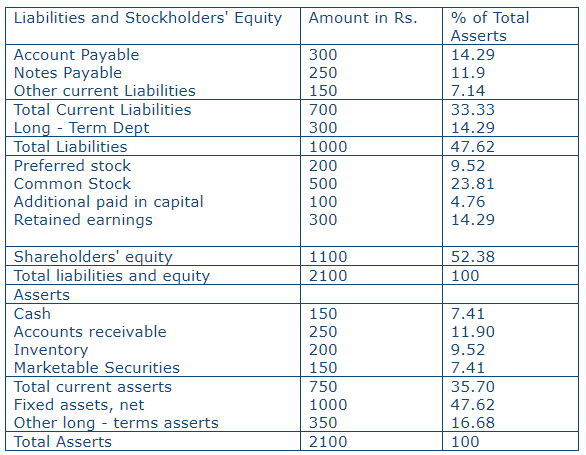Fig (1): Vertical Analysis of financial statement of Annapurna Textile Inc. as on June 2018.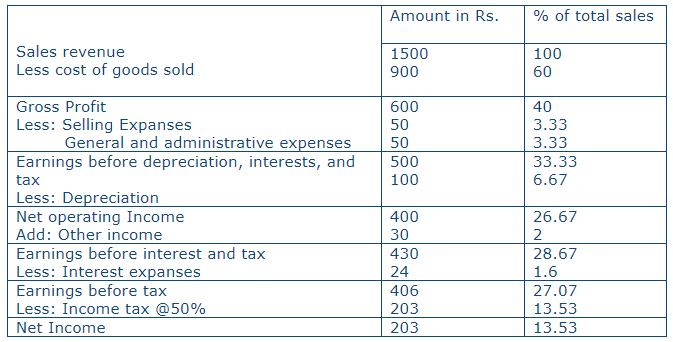Fig (2): Vertical analysis of income statement of Annapurna Textile Inc. for the year June 2018

A closer look into vertical analysis in fig (1) shows the distribution pattern of liabilities among current liabilities, long - terms liabilities and equity capital. Similarly, it shows the distribution pattern of total asserts among current asserts, fixed assets and other asserts. For example, in assessing the liquidity position of Annapurna Textile Inc. the balance sheet reveals that its total current assets represents 33.7 percentage of the total asserts of which 9.52 percentage of total assets are in the form of investment in inventory.

The Vertical Analysis income statement Fig (2) reveals what portion of sales has been absorbed by various costs, and expenses incurred and the percentage of the total sales that remains as net income. For example, the table shows that 60 percent of total sales are incurred as cost of goods sold and only 13.54 percentage of total sales are in the form of net income to the firm.

2. Horizontal Analysis

The technique of Horizontal analysis involves preparation of comparative balance sheet and comparative income statement so as to highlight changes in the various assets, liabilities income and expenditure, and the resulting profit or loss. The changes may be expressed in absolute amounts or percentages (Smart, Megginson, & Gitman, 2007). The data may be presented for two years or for a number of successive years so as to examine the trend.

As a financial statement, balance sheet is concerned with summarizing assert owned by the firm and sources of borrowing and owned funds in acquiring these assets. Figure (3) shows a hypothetical balance sheet of Annapurna Textile Inc. as on June 2018.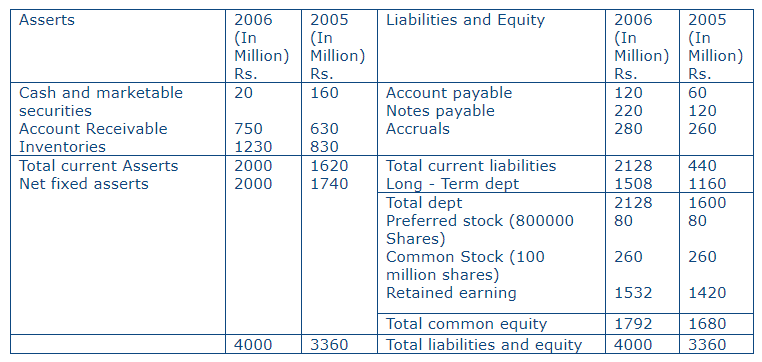Fig (3): Annapurna Textile Inc. Balance sheet on Dec 2006 (In million)

The left hand side of the balance sheet shows asserts of Annapurna Textile Inc. whereas the right hand side shows the liabilities and equity as on Dec 2006. In the above balance sheet, the assets are arrange in order of their convertibility into cash and liabilities and equity are arranged in order of their maturity. But, they can be ordered in reverse order. The proper interpretation of financial statement requires a clear and correct understanding of the basic divisions of balance sheet.

Finally, this technique involves preparation of Comparative Balance Sheet and Comparative Income Statement so as to highlight the changes in the various assets, liabilities, income and expenditure. In the Comparative Balance Sheet, the figures (3) of assets and liabilities are set out as at the beginning and at the June of the year along with the extent of increases or decreases between the two dates.

The Comparative Income Statement is drawn on the same principle as the Horizontal Balance Sheet. The statement is prepared in a vertical form. There are columns, as in a comparative balance sheet, to show the amount of income and expenditure for two years in (2005 and 2006) or more along with the increase or decrease in amounts as also percentage increases or decreases. The percentages reflects the changes that have occurred over successive periods. Unusual changes can thus be detected and their causes determined.

References
Milbourn, G., & Haight, T. (2005). Providing students with an overview of financial statements using the Dupont analysis approach. Journal of American Academy of Business, 6(1) , 46-50.

Ott, S., Riddiough, T., & Yi, H. (2009). Finance, investment and investment performance: Evidence from the REIT sector. Real Estate Economics, 33(1) , 203-207.

Smart, S., Megginson, W., & Gitman, L. (2007). Corporate Finance.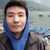Analysis are done to understand what is going on in a company. It helps investors analyze and ascertain whether the company has had consistent growth over the years and if they are utilizing fund available in a balanced way. The horizontal analysis as the name suggest is the analysis done on horizontal basis for the same item of a company’s financial statements generally for two or more years. It analyses the trend of the company by calculating the change percentage between the same line item for various years. On the other hand the vertical analysis is done by comparing the line items vertically in a financial statement with the total of either sales (in income statement) or assets (in balance sheet). This is done for single year, analyses the changes over time and the effect of one line item to another as well as to the base amount (either total revenue or total assets).

Horizontal Analysis

Horizontal analysis is the comparison of historical financial information over a series of reporting periods, or of the ratios derived from this financial information. The intent is to see if any numbers are unusually high or low in comparison to the information for bracketing periods, which may then trigger a detailed investigation of the reason for the difference. (Bragg, 2017)

Let us understand this analysis with the help of the following balance sheet.

Table 1: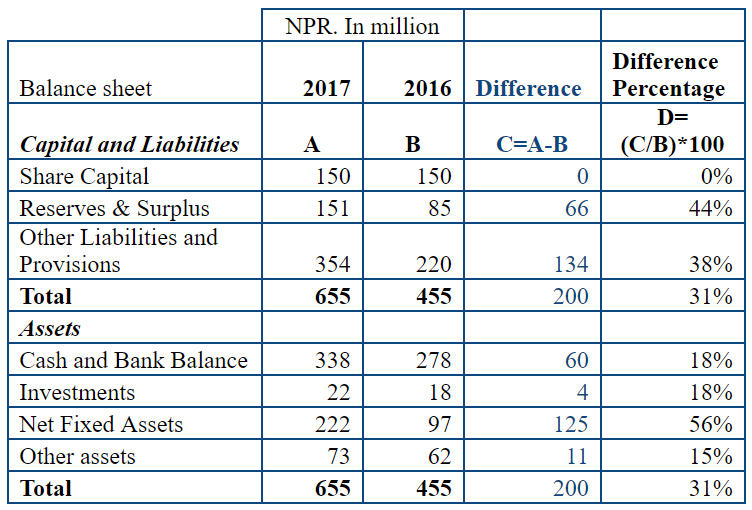Form the table above we can understand that there was no change in the share capital but the reserve and surplus was increased by 44%. Other liabilities increased by 38%, liquidity increased by 18%, investment, net fixed asset and other assets by 18%, 56% and 15% respectively. This shows there was a healthy growth in all the asset side.

Vertical Analysis.

"The product of a vertical analysis is generally referred to as a common-size statement. Common-size balance sheets bring the composition of assets and liabilities into focus, while common-size income statements highlight the relationship between expense items and sales (Mautz Jr & Angell, 2006)”.

Let us take an example for vertical analysis.

Table 2: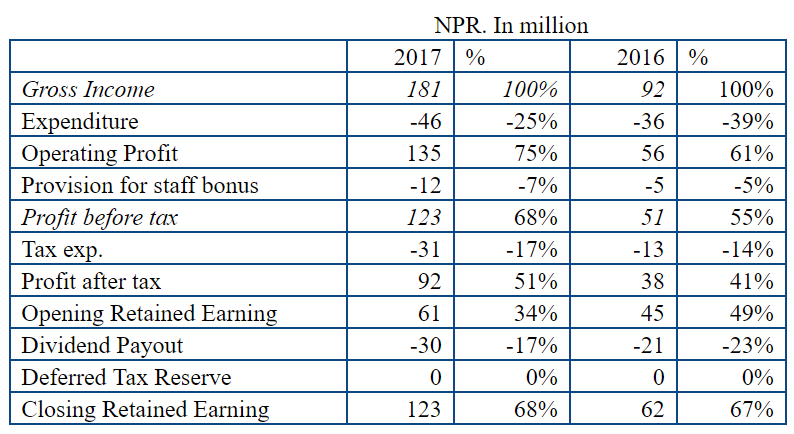Note: The percentage has been calculated by dividing the line item by total revenue and multiplying it by 100.

From table two we see that at 92 million sales, the cost of capital was 36 million, and with increase in revenue in year 2017 to 181 million, the cost of capital also decreased from 39% to 25% which show good cost management of the company. We can similarly analyze other aspects such as, dividend payout has increased from 21 million to 30 million, an increase by 23%. The retained earning has also increased from 67% to 68%. The income statement with the help of vertical analysis has helped understand that the company has performed well as compared to previous year.

In this way horizontal and vertical analysis helps to analyze the trend of a company and the income statement based on the total revenue. Based on the above analysis we see that the sales has increased resulting in increase in retained earning and dividend payout. The liquidity has also increased along with decrease in cost of capital. Although there is increase in liabilities and provision, investments in made in fixed assets and other assets have increased showing a good balance in the company statement.

References

Bragg, S. (2017, August 16). ACCOUNTING CPE COURSES & BOOKS. Retrieved from AccountingTools: accountingtools.com/articles/2017/...

Mautz Jr, D. R., & Angell, R. J. (2006). Understanding the Basics of Financial Statement Analysis. Commercial Lending Review , 27-34. Retrieved from proxy.lirn.net/MuseProxyID=mp03/Mu...